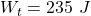## To pull a 57 kg crate across a horizontal frictionless floor, a worker applies a force of 230 N, directed 34° above the horizontal. As the c

Question

To pull a 57 kg crate across a horizontal frictionless floor, a worker applies a force of 230 N, directed 34° above the horizontal. As the crate moves 1.1 m, what work is done on the crate by (a) the worker’s force, (b) the gravitational force on the crate, and (c) the normal force on the crate from the floor? (d) What is the total work done on the crate?

in progress 0
6 months 2021-08-14T00:47:55+00:00 1 Answers 4 views 0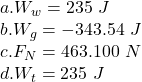Explanation:

Given: that,

Angle of inclination of the surface,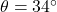mass of the crate,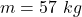Force applied along the surface,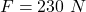distance the crate moves after the application of force,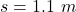a) work done = F× s

work done = 230 × 1.1

work done = 253 J

b) Work done by the gravitational force: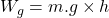where:

g = acceleration due to gravity

h = the vertically downward displacement

Now, we find the height: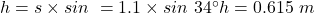So, the work done by the gravity: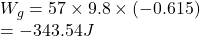∵direction of force and displacement are opposite.

= – 343.54J

c)

The normal reaction force on the crate by the inclined surface: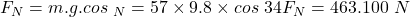d)

Total work done on crate is with respect to the worker: